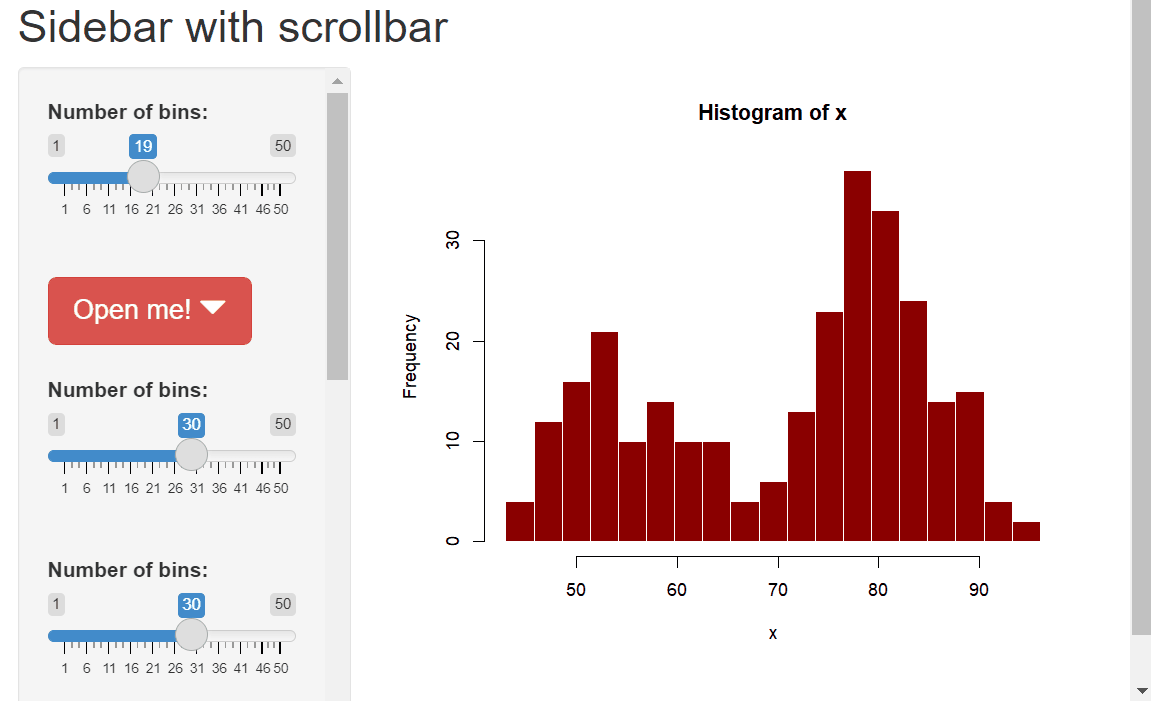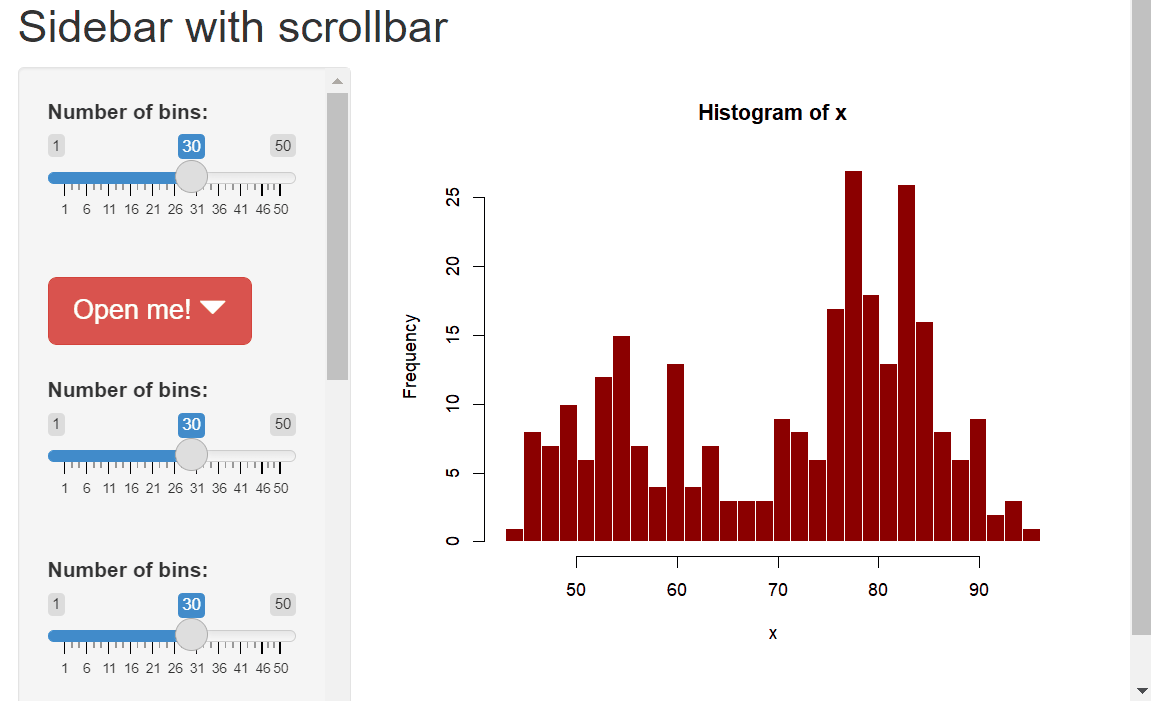Want to share your content on R-bloggers? click here if you have a blog, or here if you don't.

It is easy to have a scrollbar for the sidebar of a Shiny app: one just has to use the CSS `height: 90vh; overflow-y: auto;`:

```library(shiny)
library(shinyWidgets)

ui <- fluidPage(
titlePanel("Sidebar with scrollbar"),
sidebarLayout(
sidebarPanel(
style = "height: 90vh; overflow-y: auto;",
sliderInput("bins", "Number of bins:", min = 1, max = 50, value = 30),
br(),
dropdown(
tags\$h1("A very large dropdown"),
status = "danger",
size   = "lg",
label  = "Open me!",
width  = "500px"
),
br(),
sliderInput("bins2", "Number of bins:", min = 1, max = 50, value = 30),
br(),
sliderInput("bins3", "Number of bins:", min = 1, max = 50, value = 30),
br(),
sliderInput("bins4", "Number of bins:", min = 1, max = 50, value = 30),
br(),
sliderInput("bins5", "Number of bins:", min = 1, max = 50, value = 30),
br(),
sliderInput("bins6", "Number of bins:", min = 1, max = 50, value = 30),
br(),
sliderInput("bins7", "Number of bins:", min = 1, max = 50, value = 30),
),

mainPanel(
plotOutput("distPlot")
)

)
)

server <- function(input, output) {
output[["distPlot"]] <- renderPlot({
x    <- faithful[, 2]
bins <- seq(min(x), max(x), length.out = input[["bins"]] + 1)
hist(x, breaks = bins, col = "darkred", border = "white")
})
}

shinyApp(ui = ui, server = server)```But, as you can see, there is an issue with the `shinyWidgets::dropdown`: when one opens it, the part outside the sidebar is hidden. Here is a JavaScript solution for this issue:

```library(shiny)
library(shinyWidgets)

js <- '
\$("[id^=sw-content-]").on("shown", function(){
\$(".sidebar").css({"overflow-y": "visible"});
}).on("hidden", function(){
\$(".sidebar").css({"overflow-y": "auto"});
});
});
'

ui <- fluidPage(
titlePanel("Sidebar with scrollbar"),
sidebarLayout(
sidebarPanel(
class = "sidebar",
style = "height: 90vh; overflow-y: auto;",
sliderInput("bins", "Number of bins:", min = 1, max = 50, value = 30),
......```I find the native HTML scrollbars a bit ugly. It is possible to customize them: Styling scrollbars in CSS.